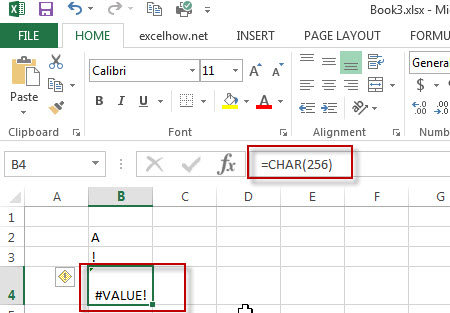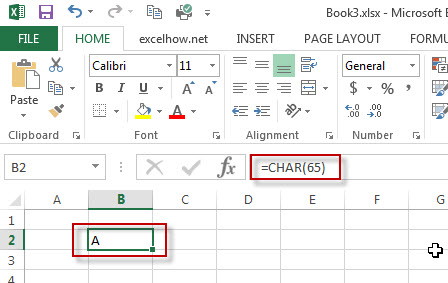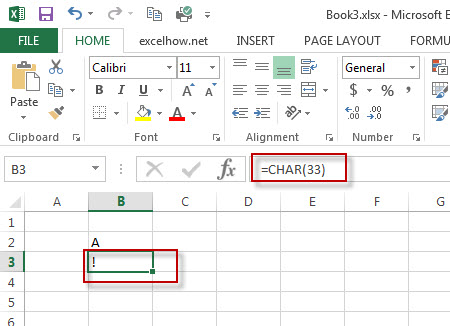# Excel CHAR Function

This post will guide you how to use Excel CHAR function with syntax and examples in Microsoft excel.

### Description

The Excel CHAR function returns the character specified by a number (ASCII Value)

The CHAR function is a build-in function in Microsoft Excel and it is categorized as a Text Function.

The CHAR function is available in Excel 2016, Excel 2013, Excel 2010, Excel 2007, Excel 2003, Excel XP, Excel 2000, Excel 2011 for Mac.

### Syntax

The syntax of the CHAR function is as below:
=CHAR(number)

Where the CHAR function argument is:

Number -This is a required argument. the number value is a ASCII value between 1 and 255.
Note: If you provided invalided number, such as: the number value is: <1 or >255, you will get an error from the excel CHAR function, it is like to be the “#VALUE!” error.### Example:

#1 get a character from number 65, 33,  in the computer’s character set### More Excel Char Function Examples

• Generate Random Character Strings
To generate random character strings in excel, you should be generate random numbers, letters, and special characters. We have talked that how to generate a random number string with Excel RANDBETWEEN function in excel…